# Creating Worksheet Functions — in Excel VBA

## In This Chapter

► Knowing why custom worksheet functions are so useful
► Exploring functions that use various types of arguments
► Understanding the Paste Function dialog box
For many people, VBA’s main attraction is the capability to create custom worksheet functions — functions that look, work, and feel just like those that Microsoft built into Excel. A custom function offers the added advantage of working exactly how you want it to. I introduce custom functions in Chapter 5. In this chapter, I get down to the nitty-gritty and describe some tricks of the trade.

## Why Create Custom Functions?

You are undoubtedly familiar with Excel’s worksheet functions — even Excel novices know how to use common worksheet functions such as SUM, AVERAGE, and IF. By my count, Excel contains more than 300 predefined worksheet functions, not counting those available through add-ins, such as the Analysis ToolPak (which is included with Excel). And if that’s not enough, you can create functions by using VBA.
With all the functions available in Excel and VBA, you may wonder why you would ever need to create functions. The answer: to simplify your work. With a bit of planning, custom functions are very useful in worksheet formulas and VBA procedures. Often, for example, you can significantly shorten a formula by creating a custom function. After all, shorter formulas are more readable and easier to work with.

## What custom worksheet functions can’t do

As you develop custom functions for use in your worksheet formulas, it’s important that you understand a key point. VBA worksheet Function procedures are essentially passive. For example, code within a Function procedure cannot manipulate ranges, change formatting, or do many of the other things that are possible with a Sub procedure. An example may help.
It might be useful to create a function that changes the color of text in a cell based on the cell’s value. Try as you might, however, you can’t write such a function. It always returns an error value.
Just remember this: A function used in a worksheet formula returns a value — it does not perform actions with objects.

## Understanding VBA Function Basics

A VBA function is a procedure that’s stored in a VBA module. You can use these functions in other VBA procedures or in your worksheet formulas.
A module can contain any number of functions. You can use a custom function in a formula just as if it were a built-in function. If the function is defined in a different workbook, however, you must precede the function name with the workbook name. For example, assume you developed a function called DiscountPrice (which takes one argument), and the function is stored in a workbook named PRICING.XLS.

### this:If you want to use this function in a different workbook, enter a formula such as this: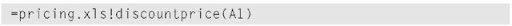If the custom function is stored in an add-in, you don’t need to precede the function name with the workbook name. I discuss add-ins in Chapter 22.
Custom functions appear in the Insert Function dialog box, in the User Defined category. The easiest way to enter a custom function into a formula is to use the Insert Function command or click the Insert Function button on the Standard toolbar. Both of these methods display the Insert Function dialog box.

## Writing Functions

Remember that a function’s name acts like a variable. The final value of this variable is the value returned by the function. To demonstrate, examine the following function, which returns the user’s first name: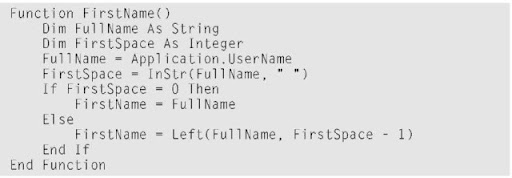This function starts by assigning the UserName property of the Application object to a variable named FullName. Next, it uses the VBA InStr function to locate the first space in the name. If there is no space, FirstSpace is equal to 0 and FirstName is equal to the entire name. If FullName does have a space, the Left function extracts the text to the left of the space and assigns it to FirstName.
Notice that FirstName is the name of the function and is also used as a variable name in the function. The final value of FirstName is the value that’s returned by the function. Several intermediate calculations may be going on in the function, but the function always returns the last value assigned to the variable that is the same as the function’s name.
All of the examples in this chapter are available at this topic’s Web site.

## Working with Function Arguments

To work with functions, you need to understand how to work with arguments. The following points apply to the arguments for Excel worksheet functions and custom VBA functions:
Arguments can be cell references, variables (including arrays), constants, literal values, or expressions.
Some functions have no arguments.
Some functions have a fixed number of required arguments (from 1 to 60). Some functions have a combination of required and optional arguments.

## Function Examples

The examples in this section demonstrate how to work with various types of arguments.

## A function with no argument

Like Sub procedures, Function procedures need not have arguments. For example, Excel has a few built-in worksheet functions that don’t use arguments, including RAND, TODAY, and NOW.
Here’s a simple example of a function with no arguments. The following function returns the UserName property of the Application object. This name appears in the Options dialog box (General tab). This simple but useful example shows the only way you can get the user’s name to appear in a worksheet formula: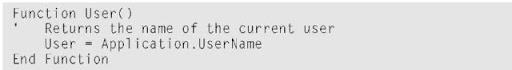When you enter the following formula into a worksheet cell, the cell displays the current user’s name: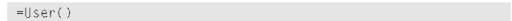As with the Excel built-in functions, you must include a set of empty parentheses when using a function with no arguments.

## A function with one argument

A single-argument function is designed for sales managers who need to calculate the commissions earned by their salespeople. The commission rate depends on the monthly sales volume; those who sell more earn a higher commission rate. The function returns the commission amount based on the monthly sales (which is the function’s only argument — a required argument). The calculations in this example are based on Table 21-1.

 Table 21-1 Commission Rates by Sales Monthly Sales Commission Rate \$0-\$9,999 8.0% \$10,000-\$19,999 10.5% \$20,000-\$39,999 12.0% \$40,000+ 14.0%

You can use several approaches to calculate commissions for sales amounts entered into a worksheet. You could write a lengthy worksheet formula such as this: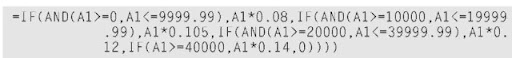A couple reasons make this a bad approach. First, the formula is overly complex. Second, the values are hard-coded into the formula, making the formula difficult to modify if the commission structure changes.
A better approach is to create a table of commission values and use a LOOKUP table function to compute the commissions: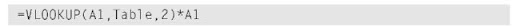Another approach, which doesn’t require a table of commissions, is to create a custom function: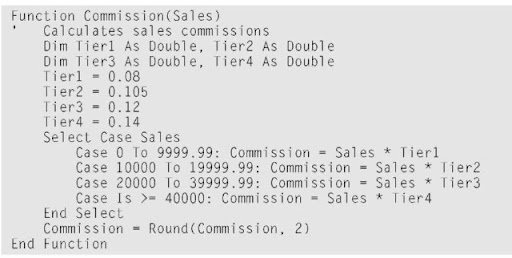After you define this function in a VBA module, you can use it in a worksheet formula. Entering the following formula into a cell produces a result of 3,000. The amount of 25000 qualifies for a commission rate of 12 percent: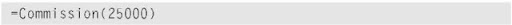Figure 21-1 shows a worksheet that uses this new function.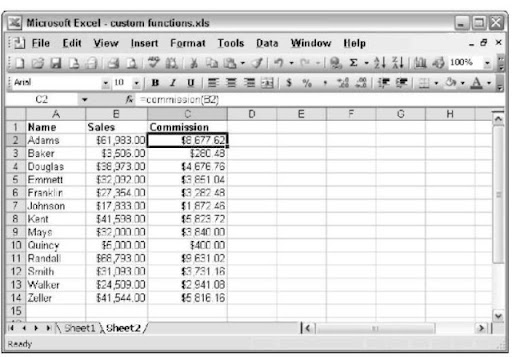Figure 21-1:
Using the Commission function in a worksheet.

## A function with two arguments

The next example builds on the preceding one. Imagine that the sales manager implements a new policy: The total commission paid increases by 1 percent for every year the salesperson has been with the company.
I modified the custom Commission function (defined in the preceding section) so that it takes two arguments, both of which are required arguments. Call this new function Commission2: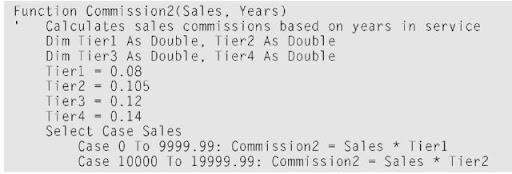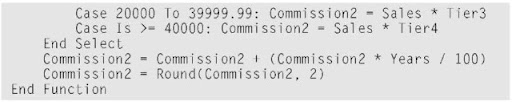I simply added the second argument (Years) to the Function statement and included an additional computation that adjusts the commission before exiting the function. This additional computation multiplies the original commission by the number of years in services, divides by 100, and then adds the result to the original computation.
Here’s an example of how you can write a formula by using this function.
(It assumes that the sales amount is in cell A1; cell B1 specifies the number of years the salesperson has worked.)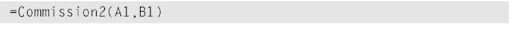## A function with a range argument

Using a worksheet range as an argument is not at all tricky; Excel takes care of the behind-the-scenes details.
Assume that you want to calculate the average of the five largest values in a range named Data. Excel doesn’t have a function that can do this, so you would probably write a formula: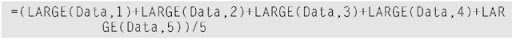This formula uses Excel’s LARGE function, which returns the nth largest value in a range. The formula adds the five largest values in the range named Data and then divides the result by 5. The formula works fine, but it’s rather unwieldy. And what if you decide that you need to compute the average of the top six values? You would need to rewrite the formula — and make sure that you update all copies of the formula.
Wouldn’t this be easier if Excel had a function named TopAvg? Then you could compute the average by using the following (nonexistent) function: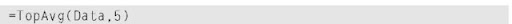This example shows a case in which a custom function can make things much easier for you. The following custom VBA function, named TopAvg, returns the average of the N largest values in a range: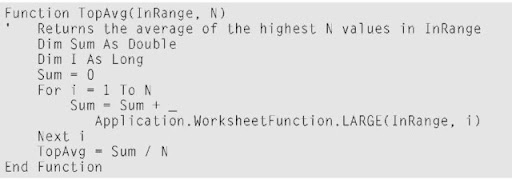This function takes two arguments: InRange (which is a worksheet range) and N (the number of values to average). It starts by initializing the Sum variable to 0. It then uses a For-Next loop to calculate the sum of the N largest values in the range. Note that I use the Excel LARGE function within the loop. Finally, TopAvg is assigned the value of Sum divided by N.
You can use all Excel worksheet functions in your VBA procedures except those that have equivalents in VBA. For example, VBA has a Rnd function that returns a random number. Therefore, you can’t use the Excel RAND function in a VBA procedure.

## A function with an optional argument

Many Excel built-in worksheet functions use optional arguments. An example is the LEFT function, which returns characters from the left side of a string. Its official syntax follows: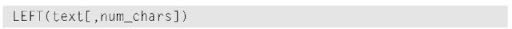The first argument is required, but the second is optional. If you omit the optional argument, Excel assumes a value of 1. Therefore, the following formulas return the same result: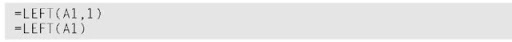The custom functions you develop in VBA also can have optional arguments. You specify an optional argument by preceding the argument’s name with the keyword Optional, followed by an equal sign and the default value. If the optional argument is missing, the code uses the default value.

## Debugging custom functions

Debugging a Function procedure can be a bit more challenging than debugging a Sub procedure. If you develop a function for use in worksheet formulas, you find that an error in the Function procedure simply results in an error display in the formula cell (usually #VALUE!). In other words, you don’t receive the normal runtime error message that helps you locate the offending statement.
You can choose among three methods for debugging custom functions:
Place MsgBox functions at strategic locations to monitor the value of specific variables. Fortunately, message boxes in Function procedures pop up when you execute the procedure. Make sure that only one formula in the worksheet uses your function, or the message boxes appear for each formula that’s evaluated — which could get very annoying.
Test the procedure by calling it from a Sub procedure. Run-time errors appear normally in a pop-up window, and you can either correct the problem (if you know it) or jump right into the debugger.
Set a breakpoint in the function and then use the Excel debugger to step through the function. You can then access all of the usual debugging tools. Refer to Chapter 13 to find out about the debugger.

### The following example shows a custom function using an optional argument: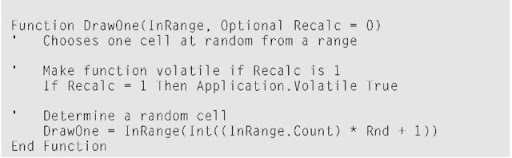This function randomly chooses one cell from an input range. The range passed as an argument is actually an array, and the function selects one item from the array at random. If the second argument is 1, the selected value changes whenever the worksheet is recalculated. (The function is made volatile.) If the second argument is 0 (or is omitted), the function is not recalculated unless one of the cells in the input range is modified.
You can use this function for choosing lottery numbers, selecting a winner from a list of names, and so on.

## A function with an indefinite number of arguments

Some Excel worksheet functions take an indefinite number of arguments. A familiar example is the SUM function, which has the following syntax: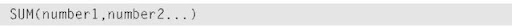The first argument is required, but you can have as many as 29 additional arguments. Here’s an example of a SUM function with four range arguments: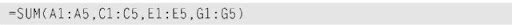Here’s a function that can have any number of single-value arguments. This function doesn’t work with multicell range arguments.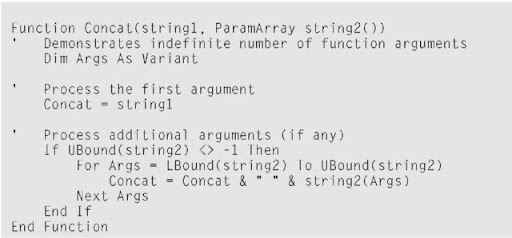This function is similar to the Excel CONCATENATE function, which combines text arguments into a single string. The difference is that this custom function inserts a space between each pair of concatenated strings.
The second argument, string2( ), is an array preceded by the ParamArray keyword. If the second argument is empty, the UBound function returns -1 and the function ends. If the second argument is not empty, the procedure loops through the elements of the string2 array and processes each additional argument. The LBound and UBound functions determine the beginning and ending elements of the array. The beginning element is normally 0 unless you either declare it as something else or use an Option Base 1 statement at the beginning of your module.
ParamArray can apply to only the last argument in the procedure. It is always a variant data type, and it is always an optional argument (although you don’t use the Optional keyword). Figure 21-2 shows this function in use. Examine the figure to see how the results differ from those produced by the Excel Concatenate function, which doesn’t insert a space between the concatenated items.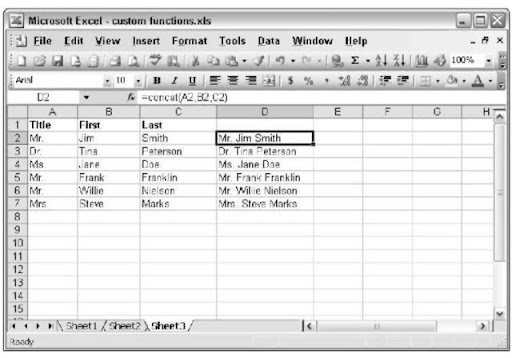Figure 21-2:
Using the Concat function.

## Using the Insert Function Dialog Box

The Excel Insert Function dialog box is a handy tool that lets you choose a worksheet function from a list and prompts you for the function’s arguments. And, as I note earlier in this chapter, your custom worksheet functions also appear in the Insert Function dialog box. Custom functions appear in the User Defined category.
Function procedures defined with the Private keyword do not appear in the Insert Function dialog box. Therefore, if you write a Function procedure that’s designed to be used only by other VBA procedures (but not in formulas), you should declare the function as Private.

## Displaying the function’s description

The Insert Function dialog box displays a description of each built-in function. But, as you can see in Figure 21-3, a custom function displays the following text as its description: No help available.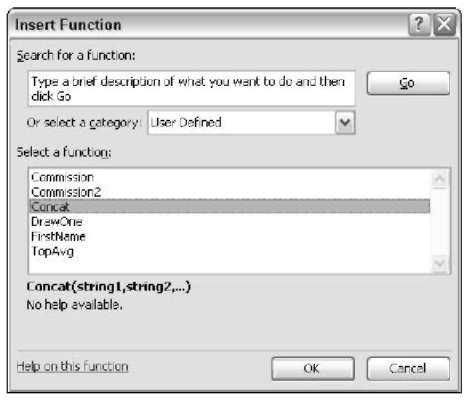Figure 21-3:
By default, the Insert Function dialog box does not provide a description for custom functions.

### To display a meaningful description of your custom function in the Paste Function dialog box, perform a few additional, (nonintuitive) steps:

1. Activate a worksheet in the workbook that contains the custom function.
2. Choose Tools Macro Macros (or press Alt+F8). The Macro dialog box appears.
3. In the Macro Name field, type the function’s name.
Note that the function does not appear in the list of macros; you must type the name in.
4. Click the Options button.
The Macro Options dialog box appears.
5. In the Description field, type a description for the function.
6. Click OK.
7. Click Cancel.
Now the Paste Function dialog box displays the description for your function; see Figure 21-4.

## Function categories

Custom functions are always listed under the User Defined category. You cannot directly create a new function category for your custom functions.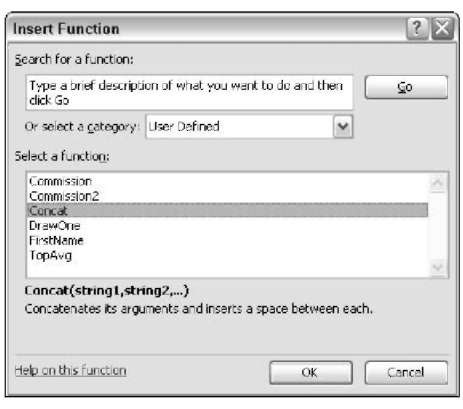Figure 21-4:
The custom function now displays a description.

## Argument descriptions

When you access a built-in function from the Insert Function dialog box, the Function Arguments dialog box displays a description of each argument. (See Figure 21-5.) Unfortunately, you can’t provide such descriptions for custom functions. You can, however, make your argument names descriptive — which is a good idea.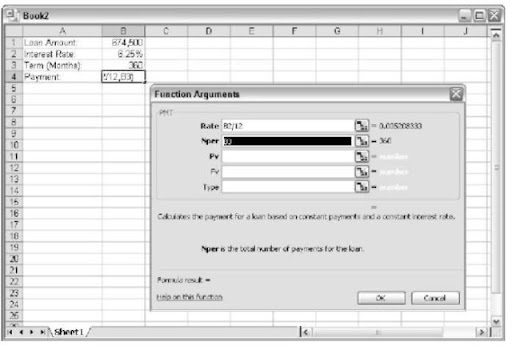Figure 21-5:
The Function Arguments dialog box displays function argument descriptions for built-in functions only.
This chapter provides lots of information about creating custom worksheet functions. Use these examples as models when you create functions for your own work. As usual, the online help provides additional details. See the next chapter if you want to find out how to make your custom functions more accessible by storing them in an add-in.

Next post:

Previous post: